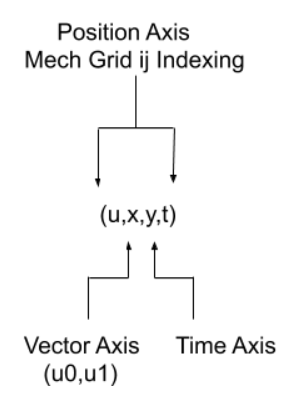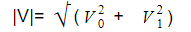Related Tags

python
community creator

# How to PyPostPiv in Python

Bindu Sanjana T

## Fluid mechanics

Fluid mechanics is a branch of physics concerned with the mechanics of fluids and the forces on them. It has applications in a variety of disciplines, including mechanical, civil, chemical, biomedical engineering, geophysics, oceanography, meteorology, astrophysics, and biology.

Applications:

1. Wind energy harvesting
2. Vechile drag reduction

## Particle Image Velocimetry(PIV)

PIV takes very tightly spaced images in time and uses some correlation between the images to calculate how far the particles have moved (this is usually done in software). The result is a set of vector fields; so, the end result gives you the velocity vectors at different speeds, different points in space, and different points in time.

### PIV Post-processing with Python

Numpy + ndarrayPython helps find the trike or what the fluid is doing by processing the vector fields.

The way to handle this in Python is with the classic scientific package numpy because it stores information in n-dimensional arrays.

Set up the numpy array so that each spatial dimension corresponds to one dimension in a numpy array – you will have one last dimension that represents the different velocity vector components.

numpy can be used to directly compute different quantities on these vector fields, but it doesn’t actually store the times at which they were captured or the actual spatial dimension, even though this information is really important for computing various mathematical quantities (e.g., spatial derivatives and time derivatives).

## PyPostPIV- A package for PIV post-processing

The PyPostPIV package is built on top of core scientific python packages:

• numpy
• scipy

The package includes:

1. Class describing field data
• Class Field2D(np.ndarray)

• PIV specific attributes (x,y, time locations)

1. Functions operating on Field2D
• Core math functions

• Other more advanced functions, i.e., turbulent kinetic energy and shear stress)

1. Visualization tools

## How to use PyPostPIV

1. Load PIV field data as Field2D instance

2. Call post-processing functions on the data

3. Visualize the data

4. For the functions that don’t exist:

• The Field2D class can be manipulated directly (since it inherits from numpy) along with core functions to make analysis intuitive

• Focus on physics by directly translating mathematics to code

import pypostpiv as ppp
field3=ppp.convert_vc7('../test3',0.01)
field3.save('test3.hdf5')


Example- Velocity Magnitudeimport pypostpiv as ppp
import numpy as np
vel_magnitude = np.sqrt(vel.u(0)**2 + vel.u(1)**2)
vel_magnitude = ppp.basics.mag(vel)


RELATED TAGS

python
community creator

CONTRIBUTOR

Bindu Sanjana T
RELATED COURSES

View all Courses

Keep Exploring

Learn in-demand tech skills in half the time